RRB JE CE (CBT I) Mock Test- 3

# RRB JE CE (CBT I) Mock Test- 3 - Civil Engineering (CE)

Test Description

## 100 Questions MCQ Test RRB JE Mock Test Series for Civil Engineering (CE) - RRB JE CE (CBT I) Mock Test- 3

RRB JE CE (CBT I) Mock Test- 3 for Civil Engineering (CE) 2023 is part of RRB JE Mock Test Series for Civil Engineering (CE) preparation. The RRB JE CE (CBT I) Mock Test- 3 questions and answers have been prepared according to the Civil Engineering (CE) exam syllabus.The RRB JE CE (CBT I) Mock Test- 3 MCQs are made for Civil Engineering (CE) 2023 Exam. Find important definitions, questions, notes, meanings, examples, exercises, MCQs and online tests for RRB JE CE (CBT I) Mock Test- 3 below.
Solutions of RRB JE CE (CBT I) Mock Test- 3 questions in English are available as part of our RRB JE Mock Test Series for Civil Engineering (CE) for Civil Engineering (CE) & RRB JE CE (CBT I) Mock Test- 3 solutions in Hindi for RRB JE Mock Test Series for Civil Engineering (CE) course. Download more important topics, notes, lectures and mock test series for Civil Engineering (CE) Exam by signing up for free. Attempt RRB JE CE (CBT I) Mock Test- 3 | 100 questions in 90 minutes | Mock test for Civil Engineering (CE) preparation | Free important questions MCQ to study RRB JE Mock Test Series for Civil Engineering (CE) for Civil Engineering (CE) Exam | Download free PDF with solutions
 1 Crore+ students have signed up on EduRev. Have you?
RRB JE CE (CBT I) Mock Test- 3 - Question 1

### D and E are the mid-points of AB and AC of ∆ABC, BC is produced to any point P; DE, DP and EP are joined. then, area of:

Detailed Solution for RRB JE CE (CBT I) Mock Test- 3 - Question 1
(By mid-point theorem)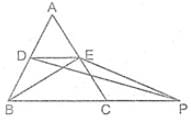DE ∥ BC

DE = 1/2 BC

⇒ (∆BDE) = 1/4 × (∆ABC)

And ∆BDE = ∆PED

[ ∵ both triangles lie on the same base DE and between two parallel lines DE and BP.]

∴ (∆PED) = 1/4 × (∆ABC)

RRB JE CE (CBT I) Mock Test- 3 - Question 2

### One side other than the hypotenuse of right angle isosceles triangle is 6 cm. The length of the perpendicular on the hypotenuse from the opposite vertex is:

Detailed Solution for RRB JE CE (CBT I) Mock Test- 3 - Question 2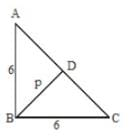Let ∠B be right angle in △ABC

and BD be perpendicular on hypotenuse AC then

1 / P2 = 1 / 62 + 1 / 62

P2 = 62 / 2 => p = 3√2.

RRB JE CE (CBT I) Mock Test- 3 - Question 3

### The speeds of two trains are in the ratio 3 : 4. They are going in opposite directions along parallel tracks. If each takes 3 seconds to cross a telegraph post, find the time taken by the trains to cross each other completely?

Detailed Solution for RRB JE CE (CBT I) Mock Test- 3 - Question 3
Speed of both trains = 3 : 4

×3 ↓ : ↓ ×3 → time

Length = 9m : 12m

Time = D / S = L1 + L2 / S1 + S2 = 9 + 12 / 4 + 3 = 2 / 14 + 3

= 3 Sec

RRB JE CE (CBT I) Mock Test- 3 - Question 4

A mother is 3 times faster than her daughter. If the daughter completes a piece of work in 15 days, how long will it take for both mother and daughter to complete the same work?

Detailed Solution for RRB JE CE (CBT I) Mock Test- 3 - Question 4
Daughter’s one day work = 1/15

Mother’s one day work = 1/5

Both can complete their work = 1/5 + 1/15 = (3 + 1)/15 = 4/15

Hence, required days= 15/4 days .

RRB JE CE (CBT I) Mock Test- 3 - Question 5

Study the following table carefully to answer the questions that follow.

Number of soldiers (in thousands) joining five different forces during six different years.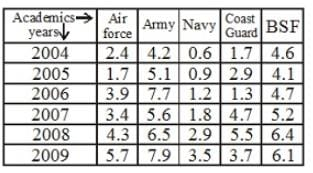Q. Total number of soldiers joining BSF in the years 2004, 2005 and 2006 was approximately what percent of the total number of soldiers joining Navy over all the years together?

Detailed Solution for RRB JE CE (CBT I) Mock Test- 3 - Question 5
Total no. of soldiers joining BSF in 2004, 2005, 2006

= 13400

Total no. of soldiers joining in Navy = 10900

Required Percentage = (13400/10900) × 100 = 123%

RRB JE CE (CBT I) Mock Test- 3 - Question 6

Study the following table carefully to answer the questions that follow.

Number of soldiers (in thousands) joining five different forces during six different years.Q. What was the ratio of the number of soldiers joining Army in the year 2008 to the number of soldiers joining coast guard in the year 2006?

Detailed Solution for RRB JE CE (CBT I) Mock Test- 3 - Question 6
Required ratio = 6.5 / 1.3 = 5 / 1 = 5 : 1
RRB JE CE (CBT I) Mock Test- 3 - Question 7

If tan (5x – 10°) = cot (5y + 20°), then the value of (x + y) is:

Detailed Solution for RRB JE CE (CBT I) Mock Test- 3 - Question 7
tan (5x – 10°) = cot (5y + 20°)

tan (5x – 10°) = tan (90° – {5y + 20°})

5x – 10° = 90° – (5y + 20°)

5x + 5y = 90° + 10° – 20°

5x + 5y = 80°

x + y = 16°

RRB JE CE (CBT I) Mock Test- 3 - Question 8

If a train, with a speed of 60 km/hr, crossed a pole in 30 second, the length of the train (in meters) is:

Detailed Solution for RRB JE CE (CBT I) Mock Test- 3 - Question 8
Speed = 60km/hr.

S = 60 × 5 / 18m/sec.= 50 / 3m⋅/sec

T = 30sec

Length = S × T = (50 / 3 × 30)m

I = 500m

RRB JE CE (CBT I) Mock Test- 3 - Question 9

The supplement of an angle is one-fourth of itself. Determine the angle and its supplement.

Detailed Solution for RRB JE CE (CBT I) Mock Test- 3 - Question 9
Let the measure of the angle be x°

Then, the measure of its supplementary angle is (180 − x). It is given that, 180− x = 1 / 4 × x

⇒ (180 − x) = x

⇒ 720 − 4x = x

⇒ 5x = 720

⇒ x = 144

Thus, the measure of the angle is 144 and the measure of its supplement 180- 144= 36

RRB JE CE (CBT I) Mock Test- 3 - Question 10

3 years ago the average age of a family of 5 members was 17 years. A baby having been born, the average age of the family is the same today. The present age of the baby is

Detailed Solution for RRB JE CE (CBT I) Mock Test- 3 - Question 10
Total age of 5 members, three years ago

= 17 × 5 = 85 years

Three years hence, Total age of 5 members = 85 + 3 x 5 = 85 + 15 = 100 years

Sum of present ages of 6 members = 17 × 6 = 102 years

Present age of baby = 102 – 100 = 2 years

RRB JE CE (CBT I) Mock Test- 3 - Question 11

A ladder leans against a vertical wall. The top of the ladder is 8 meter above the ground. When the bottom of the ladder is moved 2 meter farther away from the wall, the top of the ladder rests against the foot of the wall. What is the length of the ladder?

Detailed Solution for RRB JE CE (CBT I) Mock Test- 3 - Question 11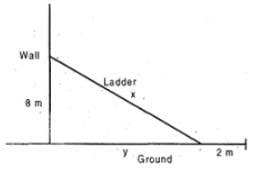Let the length of the ladder be x meter. We have

82 + y2 = x2 and (y + 2) = x

Hence, 64 + (x − 2)2 = x2

⇒ 64 + x2 − 4x + 4 = x2

⇒ 68 = 4x ⇒ x = 17 meter

RRB JE CE (CBT I) Mock Test- 3 - Question 12

The length of a Rectangular plot is decreased by 33.33%. By how much % the breadth of the plot will be increased so that the area remains constant?

Detailed Solution for RRB JE CE (CBT I) Mock Test- 3 - Question 12

33.33 = 1 / 3 → 1 / 2 = 50% [1 / 2 = 1 / 3 - 1]

RRB JE CE (CBT I) Mock Test- 3 - Question 13

A man deposited a certain amount in a fixed deposit at r % p.α., interest being compounded annually. If the interest accrued for the fourth and fifth years are Rs 13310 and Rs 14641. what is the total interest accrued for the first three years?

Detailed Solution for RRB JE CE (CBT I) Mock Test- 3 - Question 13
The interests accrued each year on compound interest form a geometric progression with 1 , as the first year interest and common ratio of (1 + r/100) Therefore, interests for 1st, 2nd, 3rd, 4th and 5th years will be

I, I(1 + r/100)1, I(1 + r/100)2, I(1 + r/100)3, I(1 + F/100)4 respectively

Given, (1 + r/100)3 = 13310

and (1 + r/100)4 = 14641

2) divided by (1), we get (1 + r/100) = 14641 / 13310 = 1.1

∴ Sum of the first three years interests = 13310 / (1.1)3 + 13310 / (1.1)2 +13310 / (1.1)1

= 13316 / 1.331 + 13316 / 2.21 + 13316 / 2.1

= 10000 + 11000 + 12100

= Rs. 33100

RRB JE CE (CBT I) Mock Test- 3 - Question 14

A person earns Rs. 5000 as an interest in 5/2 years on a certain sum invested with a company at the rate of 10% per annum. Find the sum invested by a person in the company?

Detailed Solution for RRB JE CE (CBT I) Mock Test- 3 - Question 14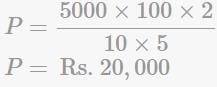RRB JE CE (CBT I) Mock Test- 3 - Question 15

The value of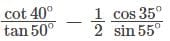is:

Detailed Solution for RRB JE CE (CBT I) Mock Test- 3 - Question 15
cot⁡θ = tan⁡(90 − θ) and cos⁡θ = sin⁡(90 − θ)

Hence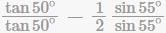1 - 1/2 = 1/2

RRB JE CE (CBT I) Mock Test- 3 - Question 16

A is twice as fast as B and B is one third as fast as C. If they together can complete work in 30 days. In how many days, A, B and C individually can do the same work?

Detailed Solution for RRB JE CE (CBT I) Mock Test- 3 - Question 16
Condition 1- A is twice as fast as

B, means their ratio is 2 : 1.

Condition 2- B is one third as fast

as C, means their ratio is 1 : 3. Then we can say, the ratio of A, B and C is as follows According to condition 1, A : B = 2 : 1

According to condition 2;

B : C = 1 : 3

Thus, Ratio of A : B : C = 2 : 1 : 3

This ratio denotes the working efficiency of A, B and C which means;

A, B and C can do = (2 + 1 + 3 = 6) work/day

Now we can calculate total work i.e.

Total work = Total days × Per day work

Total work = 30 × 6 = 180

A can do the same work in;

= (Total work / Efficiency of A) = 180 / 2 = 90 days

B can do the same work in; = (Total work / Efficiency of B)

180 / 1 = 180 days

C can do the same work in;

= (Total work / Efficiency of C) = 180 / 3 = 60 days

Thus, A, B and C can individually complete the same work in 90, 180 and 60 days, respectively.

RRB JE CE (CBT I) Mock Test- 3 - Question 17

If ab = 25, then the minimum value of a + b is:

Detailed Solution for RRB JE CE (CBT I) Mock Test- 3 - Question 17
Minimum value of (a + b) can be obtained when a = b

Then a + b = 5 + 5 (∴ ab = 25 and a = b)

= 10

RRB JE CE (CBT I) Mock Test- 3 - Question 18

If a + b + c = 1, then Find (1 + a) (1 + b) (1 + c)?

Detailed Solution for RRB JE CE (CBT I) Mock Test- 3 - Question 18
Given, a + b + c = 1 Let observe the value of a, b, c

a = 1 / 3, b = 1 / 3, c = 1 / 3

a + b + c = 1

1 / 3 + 1 / 3 + 1 / 3 = 1

3 / 3 = 1

1 = 1

put the value of a, b, c (1 + a)(1 + b)(1 + c)

(1 + 1 / 3)(1 + 1 / 3)(1 + 1 / 3)

4 / 3 × 4 / 3 × 4 / 3

64 / 27 = 2.37

RRB JE CE (CBT I) Mock Test- 3 - Question 19

The price of a shirt is ₹260 but the shopkeeper successively discount 15% & 20%. The net sales price is subject to a sales tax of 5%. What does the buyer pay?

Detailed Solution for RRB JE CE (CBT I) Mock Test- 3 - Question 19
1st discount S.P = Rs.260 − 15% Rs.260

= 260 − 39 = 221

2nd discount

S.P = Rs.221 − 20%Rs.221

= 221 − 44.2 = 176.8

Sales tax = 5 / 100 × 1768 / 10

= 8840 / 1000

= Rs⁡.8.84

= Rs.176.8 + Rs.8.84

= Rs. 185.64

RRB JE CE (CBT I) Mock Test- 3 - Question 20

A cistern from inside is 12.5 m long, 8.5 m broad,. and 4 m high and is open at the top. Find the cost of cementing the inside of a cistern at Rs. 24 per sq. m

Detailed Solution for RRB JE CE (CBT I) Mock Test- 3 - Question 20
Area of surface to be cemented = 2 x (l + b) x h + (l x b)

i.e, area of four walls + area of the floor

= 2 x (21) x 4 + (106.25)

= 274.25 m2

∴ cost of cementing = 24 x 274.25 = Rs. 6582

RRB JE CE (CBT I) Mock Test- 3 - Question 21

A goat is tied to a pole fixed at a corner outside a room with a square base in a grass field. It is tied using a 14 m long rope. The side of the base of the room is 21 m. Find the area of the field over which the goat can graze (in sq. m).

Detailed Solution for RRB JE CE (CBT I) Mock Test- 3 - Question 21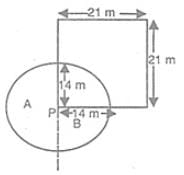Let the goat be tied at P.

The area over which the goat could graze = sum of the areas of the regions A and B = Area of a sector of radius 14 m and central angle 270°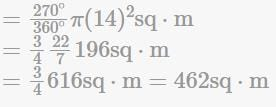RRB JE CE (CBT I) Mock Test- 3 - Question 22

An article is sold at a loss of 10%. Had it been sold for Rs. 9 more, there would have been a gain of 22 x 1/2 % on it. The cost price of the article is :

Detailed Solution for RRB JE CE (CBT I) Mock Test- 3 - Question 22
Let the cost price of the article = Rs. x

S.P. at 10% loss = Rs0.9x

According to question if it is sold by Rs 9 more there will be gain of 25 / 2%

0.9x + 9 = x ×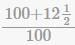0.9x + 9 = 225x / 200

⇒ 180x + 1800 = 225x

⇒ 225x − 180x = 1800

⇒ 45x = 1800

∴ x = Rs.40

Alternate solution, Let the CP of the article =100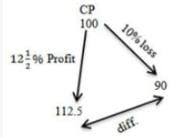x × 22.5 = 9

x = 9 / 22.5

= 18 / 45

Required, ⇒ CP × x

⇒ 100 × 18 / 45

⇒ 40

RRB JE CE (CBT I) Mock Test- 3 - Question 23

A water tank is 6 m long, 5 m broad and 3.4 m high. Find the capacity of the tank in litres?

Detailed Solution for RRB JE CE (CBT I) Mock Test- 3 - Question 23
Volume = 6 × 5 × 3.4

Volume = 102cu.cm

1cu.m = 100cu.cm

102cu.m = 102 × 100

100cu⋅cm = 1 litre

1cu⋅cm = 1 / 1000

10200cu⋅cm = (1 / 1000) × 10200

= 10.2 litres

RRB JE CE (CBT I) Mock Test- 3 - Question 24

The ratio of the area of a square to that of the square drawn on its diagonal is:

Detailed Solution for RRB JE CE (CBT I) Mock Test- 3 - Question 24
Let the side of the square be ‘α’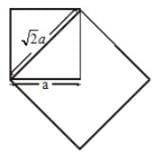Its area = a2

Area of square on the diagonal = (√2a)2 = 2a2

Required ratio = a2 / 2a2 = 1 : 2

RRB JE CE (CBT I) Mock Test- 3 - Question 25

If sec⁡θ + tan⁡θ = p, the p2 - 1 / p2 + 1 = ?

Detailed Solution for RRB JE CE (CBT I) Mock Test- 3 - Question 25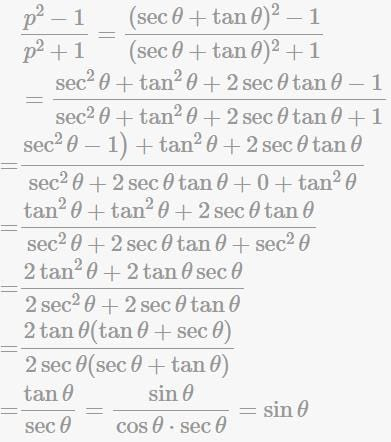RRB JE CE (CBT I) Mock Test- 3 - Question 26

In the given figure, if ∠ABC = 90°, and ∠A = 30°, then ∠ACD =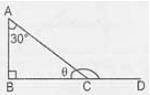Detailed Solution for RRB JE CE (CBT I) Mock Test- 3 - Question 26
∠ACD = ∠B + ∠A

= 90° + 30°

= 120° (exterior angle)

RRB JE CE (CBT I) Mock Test- 3 - Question 27

A certain sum of money yields ₹ 1261 as compound interest for 3 years at 5% per annum. The sum is

Detailed Solution for RRB JE CE (CBT I) Mock Test- 3 - Question 27
Let the principal , compound interest rate and time be x, R and T respectively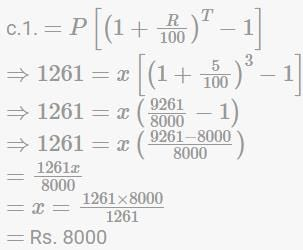RRB JE CE (CBT I) Mock Test- 3 - Question 28

If 2x - 1 + 2x + 1 = 320, then x = ?

Detailed Solution for RRB JE CE (CBT I) Mock Test- 3 - Question 28
2x − 1 + 2x + 1 = 320

2x − 1(1 + 22) = 320

2x − 1 = 320

2x − 1 = 320 / 5 = 64

2x − 1 = 26

x − 1 = 6

X = 7

RRB JE CE (CBT I) Mock Test- 3 - Question 29

The value of (sin2 25° + sin2 65°) is

Detailed Solution for RRB JE CE (CBT I) Mock Test- 3 - Question 29
sin2⁡25 + sin2⁡65 = sin2⁡25 + sin2⁡(90 − 25)

= sin2⁡25 + cos2⁡25 = 1

RRB JE CE (CBT I) Mock Test- 3 - Question 30

A is twice as good as workman as B. Together, they finish the work in 14 days. In how many days can it be done by each separately?

Detailed Solution for RRB JE CE (CBT I) Mock Test- 3 - Question 30
As per the question, A do twice the work as done by B.

So A : B = 2 : 1

Also (A + B)'s one-day work = 1 / 14

To get days in which B will finish the work,

let's calculate work done by B in 1 day

= (1 / 14) x (1 / 3) = 1 / 42

So B will finish the work in 42 days and A will finish the work in 21 days.

RRB JE CE (CBT I) Mock Test- 3 - Question 31

There are several chickens and rabbits in a cage (with no other types of animals). There are 72 heads and 200 feet inside the cage. How many chickens and rabbits are there?

Detailed Solution for RRB JE CE (CBT I) Mock Test- 3 - Question 31
No. of chicken = x

No. of rabbit = y

No. of legs = 2x + 4y = 200

x + 2y = 100 → 1

No. of heads = x + y = 72 → 2

1 - 2

y = 100 - 72 = 28

= 72 - 28 = 44

Hence the correct answer is option A

RRB JE CE (CBT I) Mock Test- 3 - Question 32

Find the next term in the given series?

BY, DW, GT, KP, ?

Detailed Solution for RRB JE CE (CBT I) Mock Test- 3 - Question 32
The first letter is increased by 2, 3, 4, 5 and the second letter is decreased by 2, 3, 4, 5. Hence the next term is K + 5, P - 5 = PK.
RRB JE CE (CBT I) Mock Test- 3 - Question 33

Read the following statements. The conclusions are given in the options, you have to read the conclusions and then decide which one of them among the options definetly does not follow.

Statements:

1. Some tables are chairs.

2. No Chair is a couch.

3. All sofas are couch.

Detailed Solution for RRB JE CE (CBT I) Mock Test- 3 - Question 33
The venn diagram of the statements are shown below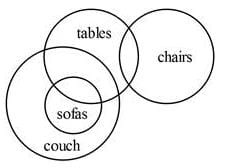All sofas are not chairs. So some sofas being not chairs is true.

So Option 1 is true.

Option 2 is also true.

Option 3 is also true.

Option 4 is false.

RRB JE CE (CBT I) Mock Test- 3 - Question 34

Which of the following will be the mirror image of the given question figure (x), if the mirror is placed along the line MN. Choose the correct mirror image among (I), (II), (III) and (IV) given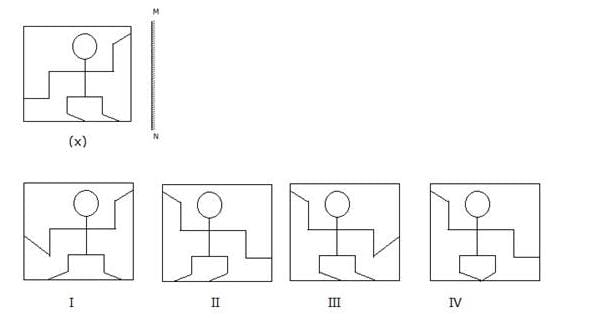Detailed Solution for RRB JE CE (CBT I) Mock Test- 3 - Question 34
Image II is the correct image of (x).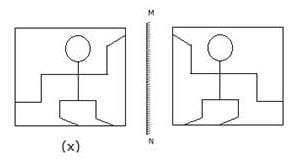RRB JE CE (CBT I) Mock Test- 3 - Question 35

If in a certain code, GLACIER is coded as 6293017 and FRUIT is coded as 47806, then how will FILTER be coded as in the same code?

Detailed Solution for RRB JE CE (CBT I) Mock Test- 3 - Question 35
Here, the letters of the word are coded as certain number. For solving this, we find out the codes for letters from the given codes of the words as follows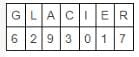And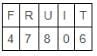So,we get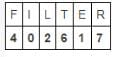RRB JE CE (CBT I) Mock Test- 3 - Question 36

Harish is standing to the north-west of Kishore. Raju is standing to the south-east of Prem. Prem is standing to the south-east of Kishore. Manu is standing to the east of Kishore. What is the direction of Raju with respect to Harish?

Detailed Solution for RRB JE CE (CBT I) Mock Test- 3 - Question 36
The positions are shown in the figure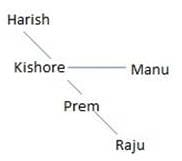Raju South-east of Harish

RRB JE CE (CBT I) Mock Test- 3 - Question 37

In the following question, select the related numbers from the given alternatives.

9246 : 1926 ∷ 3968 : ?

Detailed Solution for RRB JE CE (CBT I) Mock Test- 3 - Question 37
9 * 2 = 18 → 18 + 1 = 19

4 * 6 = 24 → 24 + 2 = 26

Becomes 1926.

Similarly,

3 * 9 = 27 → 27 + 1 = 28

6 * 8 = 48 → 48 + 2 = 50

Becomes 2850.

RRB JE CE (CBT I) Mock Test- 3 - Question 38

Arrange the given words in the sequence in which they occur in the dictionary and then choose the correct sequence.

1- Arraign 2- Arrest 3- Arrive 4- Array

Detailed Solution for RRB JE CE (CBT I) Mock Test- 3 - Question 38
The correct order is arraign, array, arrest, arrive.
RRB JE CE (CBT I) Mock Test- 3 - Question 39

In order to complete the set, which one of the following set of letters should be placed at the gaps sequentially?

_QOMKI_

Detailed Solution for RRB JE CE (CBT I) Mock Test- 3 - Question 39
The sequence is letters arranged in reverse alphabetical order, skipping a letter: S, Q, O, M, K, I, G.
RRB JE CE (CBT I) Mock Test- 3 - Question 40

A Faulty watch loses 5 minutes in 2 hours. It was set right at 6 o' clock in the morning. At 6 o' clock in the morning on the next day, what was the time shown in the faulty watch?

Detailed Solution for RRB JE CE (CBT I) Mock Test- 3 - Question 40
Time duration = 24 hours

In 2 hours, the faulty watch loses 5 minutes

In 24 hours, the faulty watch loses 60 minutes or 1 hour

Time shown in the faulty watch will be 05:00 a.m.

RRB JE CE (CBT I) Mock Test- 3 - Question 41

In a certain code, BOTANY is written as Q3M. How is RHYTHM written in that code?

Detailed Solution for RRB JE CE (CBT I) Mock Test- 3 - Question 41
In this code,

B (2) + O (15) = 17 → Q

T (20) + A (1) = 21 = 2 + 1 = 3

N (14) + Y (25) = 39 = 26 + 13 = M

So, R (18) + H (8) = 26 = Z

Y (25) + T (20) = 45 = 4 + 5 = 9

H (8) + M (13) = 21 → U

Hence, the required code is Z9U

RRB JE CE (CBT I) Mock Test- 3 - Question 42

Each question given below consists of a statement, followed by two arguments numbered I and II. Decide which of the arguments is a 'strong' argument and which is a 'weak' argument and choose the corresponding option as your answer.

Should the age of drinking be reduced from 21 years to 18 years?

I. Yes, because alcohol is not as harmful to health as smoking is.

II. Yes, because if an 18-year old adult can have the right to vote and get married, he can also have the right to drink

Detailed Solution for RRB JE CE (CBT I) Mock Test- 3 - Question 42
I is not a strong argument as the statement has not talked about smoking, which is out of scope here. II is a strong argument as both voting and marrying are rights that one gains after turning 18, so being an adult, one must also be able to drink. B is the right answer.
RRB JE CE (CBT I) Mock Test- 3 - Question 43

Each question given below consists of a statement, followed by two arguments numbered I and II. You have to decide which of the arguments is a 'strong' argument and which is a 'weak' argument.

Statement: Should luxury hotels be banned in India?

Arguments:

I. Yes. They are places from where international criminals operate.

II. No. Affluent foreign tourists will have no place to stay.

Detailed Solution for RRB JE CE (CBT I) Mock Test- 3 - Question 43
The luxury hotels are a mark of country's standard and a place for staying for the affluent foreign tourists. So, argument II holds. Argument I is not a strong reason because ban on hotel is not a way to do away with the activities of international criminals.
RRB JE CE (CBT I) Mock Test- 3 - Question 44

P remembers that his joining date in the office is after 18th October, but not on any odd-numbered date. Q remembers that P is joining in October. R, who is P's friend remembers that P is joining before 21st October. Then, on which date is P joining?

Detailed Solution for RRB JE CE (CBT I) Mock Test- 3 - Question 44
P's joining is on even numbered date between 18th and 21st, hence, 20th October.
RRB JE CE (CBT I) Mock Test- 3 - Question 45

Identify the diagram that best represents the relationship among the given classes.

Human, children, Male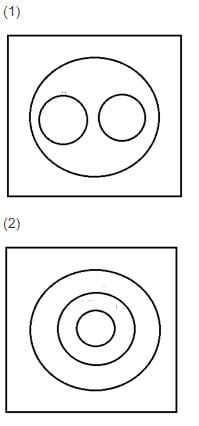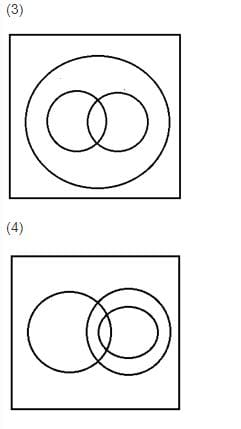Detailed Solution for RRB JE CE (CBT I) Mock Test- 3 - Question 45
All children are human, and some children are males, all males are humans, thus, 3rd shows the right relationship.
RRB JE CE (CBT I) Mock Test- 3 - Question 46

In the following question, select the odd letters from the given alternatives.

Detailed Solution for RRB JE CE (CBT I) Mock Test- 3 - Question 46
The position value of 2nd alphabet is twice as that of the 1st alphabet. The 3rd alphabet comes just after the 2nd alphabet in the alphabet series

G(7) N(14) O(15)

D(4) H(8) I(9)

I(9) R(18) S(19)

M(13) Y(25) Z(26)

RRB JE CE (CBT I) Mock Test- 3 - Question 47

Select the related letters/words/numbers from the given alternatives

AK : FP : EO : ?

Detailed Solution for RRB JE CE (CBT I) Mock Test- 3 - Question 47
In this sequence of alphabets, the difference between the numerical value of the 2 alphabets is 10 .i.e.(1, 11), (6, 16), (5, 15), (9, 19).
RRB JE CE (CBT I) Mock Test- 3 - Question 48

Find the odd one out.

Detailed Solution for RRB JE CE (CBT I) Mock Test- 3 - Question 48
8 x 4 x 2 = 64

4 x 4 x 4 = 64

2 x 8 x 4 = 64

6 x 2 x 4 = 64 not 48

RRB JE CE (CBT I) Mock Test- 3 - Question 49

In the following question, a figure (x) is given having a pattern, whose two portions marked as A and B are missing. Below the figure, four patterns (i), (ii), (iii) and (iv) are given, among which two of them fit A and B correctly. Select the option which matches both A and B correctly.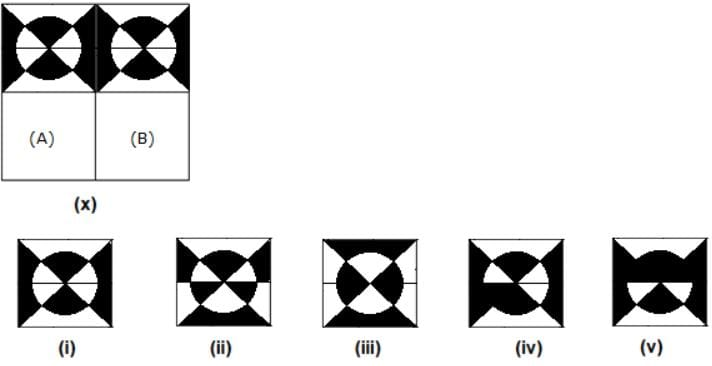Detailed Solution for RRB JE CE (CBT I) Mock Test- 3 - Question 49
Complete figure is -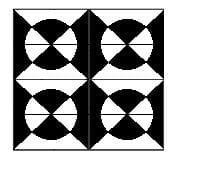RRB JE CE (CBT I) Mock Test- 3 - Question 50

How many triangles are present in the given figure?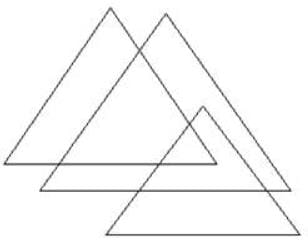Detailed Solution for RRB JE CE (CBT I) Mock Test- 3 - Question 50
There are 6 triangles in the given figure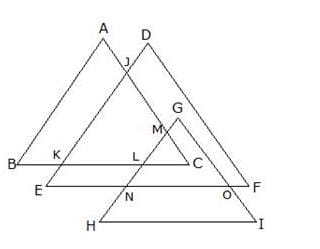Triangles are - ABC, CLM, CKJ, DEF, GHI, GNO.

RRB JE CE (CBT I) Mock Test- 3 - Question 51

Find out from amongst the four alternatives as to how the pattern would appear on the sheet when the sheet is folded at the dotted line and is punched as given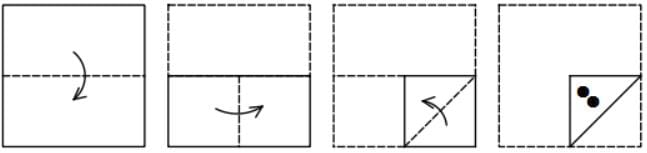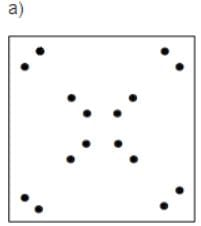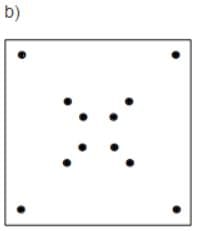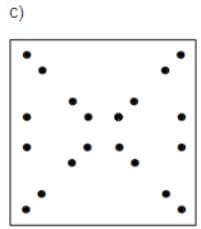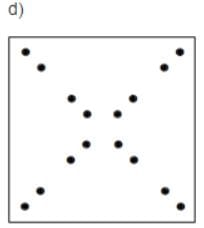Detailed Solution for RRB JE CE (CBT I) Mock Test- 3 - Question 51
The paper when opened would look like the one given in figure d
RRB JE CE (CBT I) Mock Test- 3 - Question 52

Each question given below consists of a statement, followed by two arguments numbered I and II. Decide which of the arguments is a 'strong' argument and which is a 'weak' argument and choose the corresponding option as your answer.

Should sex education be made compulsory at all schools?

I. Yes, because parents are not capable of educating their children.

II. No, because this leads students astray.

Detailed Solution for RRB JE CE (CBT I) Mock Test- 3 - Question 52
Neither of the two arguments give valid arguments in favour or against this step, which makes D the right answer.
RRB JE CE (CBT I) Mock Test- 3 - Question 53

In a queue, Tina is standing 8th from the left and 9th from the right. Pooja is to the immediate left of Sara, who is 8th towards right of Tina. What is the position of Pooja from the right side?

Detailed Solution for RRB JE CE (CBT I) Mock Test- 3 - Question 53
Number of students = Position of Tina from left + Position of Tina from right -1 = 8 + 9 - 1 = 16

Position of Sara = 8 + 8 = 16th from left

Position of Pooja = 16 - 1 = 15th from left

Position of Pooja from right = 16 - 15 + 1 = 2 from right

RRB JE CE (CBT I) Mock Test- 3 - Question 54

Three positions of a same cube are shown below. Which number appears on the face opposite to '5'?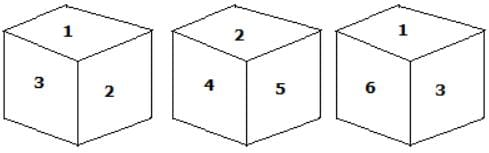Detailed Solution for RRB JE CE (CBT I) Mock Test- 3 - Question 54
'3' appears opposite to '5'.

We get: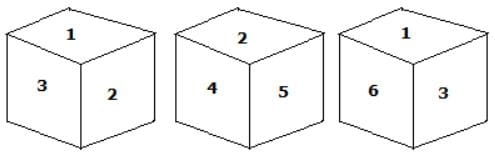RRB JE CE (CBT I) Mock Test- 3 - Question 55

C is wife of B and B is son of K. F is brother of C and A is nephew of F. J is father of F. Q is daughter of F. How is C related to K?

Detailed Solution for RRB JE CE (CBT I) Mock Test- 3 - Question 55
C is wife of B and K is mother of B. therefore, C is daughter in law of K.
RRB JE CE (CBT I) Mock Test- 3 - Question 56

National Education Day is celebrated on November _____.

Detailed Solution for RRB JE CE (CBT I) Mock Test- 3 - Question 56
11th November is National Education Day. This day is observed to commemorate the birth anniversary of Maulana Abul Kalam Azad, the first education minister of independent India, who served from 15 August 1947 until 2 February 1958.
RRB JE CE (CBT I) Mock Test- 3 - Question 57

National Science Day is celebrated every year on:

Detailed Solution for RRB JE CE (CBT I) Mock Test- 3 - Question 57
National Science Day is celebrated every year on 28 February.
RRB JE CE (CBT I) Mock Test- 3 - Question 58

India has been elected as a Member of the ________ for another 4-year term (2019-2022) recently.

Detailed Solution for RRB JE CE (CBT I) Mock Test- 3 - Question 58
India has been elected as a Member of the International Telecommunications Union (ITU) Council for another 4-year term (2019-2022). The elections to the Council were held during the ongoing ITU Plenipotentiary Conference 2018 at Dubai, UAE.
RRB JE CE (CBT I) Mock Test- 3 - Question 59

Which of the following is called a ‘Cash Crop’?

Detailed Solution for RRB JE CE (CBT I) Mock Test- 3 - Question 59
Sugarcane is a cash-crop
RRB JE CE (CBT I) Mock Test- 3 - Question 60

The third edition of Indian Ocean Conference was held in -

Detailed Solution for RRB JE CE (CBT I) Mock Test- 3 - Question 60
The third edition of Indian Ocean Conference was held in Hanoi, capital city of Vietnam from 27-28 August 2018.
RRB JE CE (CBT I) Mock Test- 3 - Question 61

What was the official language of the Sultanate?

Detailed Solution for RRB JE CE (CBT I) Mock Test- 3 - Question 61
Persian.

As soon as the Ghurids invaded northern India in the 12th century, and doubtlessly with the founding of the Delhi Sultanate. The official language of the Ghurids, Delhi Sultanate, the Mughal Empire, and their successor states, as well as the cultured language of poetry and literature, was Persian, albeit the language of religion was Arabic.

RRB JE CE (CBT I) Mock Test- 3 - Question 62

Which of the following crops are suitable for growing on black soil?

Detailed Solution for RRB JE CE (CBT I) Mock Test- 3 - Question 62
All of these crops can be grown on black soil.
RRB JE CE (CBT I) Mock Test- 3 - Question 63

The sultan who described himself as “The second Alexander”(Sikander-i-e-Sani’ ) was?

Detailed Solution for RRB JE CE (CBT I) Mock Test- 3 - Question 63
Alauddin Khilji heard of becoming second Alexander and order to engrave his name as second Alexander in his coins. He assumed the title of "Sikandar -i- Sani"
RRB JE CE (CBT I) Mock Test- 3 - Question 64

Jharia of Jharkhand is well-known for its -

Detailed Solution for RRB JE CE (CBT I) Mock Test- 3 - Question 64
Jharia is well-known for its coal mines.
RRB JE CE (CBT I) Mock Test- 3 - Question 65

Sher Shah defeated Humayun and captured Gaur in the battle of:

Detailed Solution for RRB JE CE (CBT I) Mock Test- 3 - Question 65
Sher Shah defeated Humayun in the Battle of Chausa in 1539 CE and captured Gaur.
RRB JE CE (CBT I) Mock Test- 3 - Question 66

Which one is NOT situated at Fatehpur Sikri?

Detailed Solution for RRB JE CE (CBT I) Mock Test- 3 - Question 66
Moti Masjid one is NOT situated at Fatehpur Sikri.
RRB JE CE (CBT I) Mock Test- 3 - Question 67

Tropic of Cancer does not pass through -

Detailed Solution for RRB JE CE (CBT I) Mock Test- 3 - Question 67
Tropic of Cancer does not pass through Maharashtra state.
RRB JE CE (CBT I) Mock Test- 3 - Question 68

How many National Parties are there in India(as on Jan 2019)?

Detailed Solution for RRB JE CE (CBT I) Mock Test- 3 - Question 68
There are 7 National Parties in India.
RRB JE CE (CBT I) Mock Test- 3 - Question 69

Which place is famous for diamond-mining?

Detailed Solution for RRB JE CE (CBT I) Mock Test- 3 - Question 69
Panna is famous for diamond-mining.
RRB JE CE (CBT I) Mock Test- 3 - Question 70

The longest serving Governor of the RBI is-

Detailed Solution for RRB JE CE (CBT I) Mock Test- 3 - Question 70
Holding office for over seven years, Benegal Rama Rau is the longest-serving governor, while Amitav Ghosh's 20-day term is the shortest.
RRB JE CE (CBT I) Mock Test- 3 - Question 71

Which of the following plant hormones plays main role in separation of leaves?

Detailed Solution for RRB JE CE (CBT I) Mock Test- 3 - Question 71
Abscisic acid plays main role in separation of leaves.

Florigens - blooming of flowers

Ethylene - Ripening of fruits

Cytokinin - Cell division

RRB JE CE (CBT I) Mock Test- 3 - Question 72

What is the escape velocity for moon?

Detailed Solution for RRB JE CE (CBT I) Mock Test- 3 - Question 72
Escape velocity for moon is 2.4 Km/s. For earth it is around 11.2 Km/s. Escape velocity is the speed that an object needs to be traveling to break free of a planet or moon's gravity well and leave it without further propulsion.
RRB JE CE (CBT I) Mock Test- 3 - Question 73

Which of the following falls under the category of Abiotic components of environment?

Detailed Solution for RRB JE CE (CBT I) Mock Test- 3 - Question 73
Sunlight is an example of an Abiotic component.

Abiotic factors refer to non-living physical and chemical elements in the ecosystem.

Biotic factors are living or once-living organisms in the ecosystem.

RRB JE CE (CBT I) Mock Test- 3 - Question 74

A car accelerates to a speed of 17 m/s over a distance of 25 m. The uniform acceleration of the car will be ________.

Detailed Solution for RRB JE CE (CBT I) Mock Test- 3 - Question 74
vi = 0 m/s, vf = 17 m/s

S = 25 m

vf2 = vi2 + 2as

(17)2 = (0)2 + 2(a)(25 m)

289 = 0 + (50)a

289 = (50)a

a = 289/50

a = 5.78 m/sec2

RRB JE CE (CBT I) Mock Test- 3 - Question 75

What is the mass number of Gold?

Detailed Solution for RRB JE CE (CBT I) Mock Test- 3 - Question 75
The mass number of Gold is 197. The massan number is the sum of the numbers of protons and neutrons present in the nucleus of an atom.

Mass numbers of some common elements-

Neon- 20

Sodium- 23

Silver- 108

RRB JE CE (CBT I) Mock Test- 3 - Question 76

Feldspar is an ore of _______.

Detailed Solution for RRB JE CE (CBT I) Mock Test- 3 - Question 76
Feldspar is an ore of Aluminium.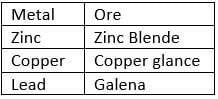RRB JE CE (CBT I) Mock Test- 3 - Question 77

_________ is a constituent of ear drop.

Detailed Solution for RRB JE CE (CBT I) Mock Test- 3 - Question 77
Boric acid is a constituent of ear drop.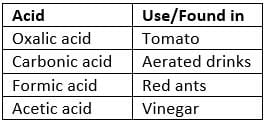RRB JE CE (CBT I) Mock Test- 3 - Question 78

_______ is not an example of monocotyledon plant.

Detailed Solution for RRB JE CE (CBT I) Mock Test- 3 - Question 78
Cabbage is not an example of a monocotyledon plant it is a dicotyledon. The Dicotyledons have two lateral cotyledons in each seed, while Monocotyledon have one cotyledon, with a terminal position.
RRB JE CE (CBT I) Mock Test- 3 - Question 79

Which of the following is often regarded as the purest form of carbon?

Detailed Solution for RRB JE CE (CBT I) Mock Test- 3 - Question 79
Diamond is often regarded as the purest form of carbon. In its purest form diamond is colourless, lustrous, Crystalline solid, It is a bad conductor of heat and electricity because it lacks free electrons. It also has a very high melting point.
RRB JE CE (CBT I) Mock Test- 3 - Question 80

Which is the second largest gland of the Human body?

Detailed Solution for RRB JE CE (CBT I) Mock Test- 3 - Question 80
After the liver the second largest gland is Pancreas
RRB JE CE (CBT I) Mock Test- 3 - Question 81

____________ states that, properties of elements are a periodic function of their atomic weight.

Detailed Solution for RRB JE CE (CBT I) Mock Test- 3 - Question 81
Mendeleev's periodic law states that properties of elements are a periodic function of their atomic weight. Modern periodic law-The physical and chemical properties of elements are periodic functions of their atomic number. Newlands's law of octaves-if the chemical elements are arranged according to increasing atomic weight, those with similar physical and chemical properties occur after each interval of seven elements.
RRB JE CE (CBT I) Mock Test- 3 - Question 82

____________ is the process in which a material changes from a frozen solid to a gas without passing through the intermediate liquid state.

Detailed Solution for RRB JE CE (CBT I) Mock Test- 3 - Question 82
• Sublimation is the process in which a material changes from a frozen solid to a gas without passing through the intermediate liquid state.

• Condensation is the process by which water vapor in the air is changed into liquid water.

• Evaporation is the process by which water changes from a liquid to a gas or vapor.

• Decantation is a process to separate mixtures by removing a liquid layer that is free of a precipitate.

RRB JE CE (CBT I) Mock Test- 3 - Question 83

Which of the following statements in the context of electromagnetic waves is/are not true?

Detailed Solution for RRB JE CE (CBT I) Mock Test- 3 - Question 83
• Electromagnetic waves propagate with the speed of light.

• Transmission of energy through a vacuum or using no medium is accomplished by electromagnetic waves. It is caused by the oscillation of electric and magnetic fields.

RRB JE CE (CBT I) Mock Test- 3 - Question 84

Kelvin is an SI Unit of ________.

Detailed Solution for RRB JE CE (CBT I) Mock Test- 3 - Question 84
The SI Unit of temperature is Kelvin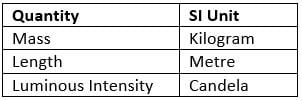RRB JE CE (CBT I) Mock Test- 3 - Question 85

Which of the following is a water-soluble vitamin?

Detailed Solution for RRB JE CE (CBT I) Mock Test- 3 - Question 85
• Vitamin B is a water-soluble vitamin. A vitamin that can dissolve in water. Water-soluble vitamins are carried to the body's tissues but are not stored in the body.

• Vitamin A, D, E & K are fat soluble vitamins.

RRB JE CE (CBT I) Mock Test- 3 - Question 86

One mole of oxygen atoms contains __________ oxygen atoms.

Detailed Solution for RRB JE CE (CBT I) Mock Test- 3 - Question 86
• One mole of oxygen atoms contains 6.023 X 1023 oxygen atoms.

• A mole corresponds to the mass of a substance that contains 6.023 x 1023 particles of the substance. The mole is the SI unit for the amount of a substance and its symbol is mol.

• Definition of mole- 1 mol of carbon-12 has a mass of 12 grams and contains 6.022140857 x 1023 of carbon atoms.

RRB JE CE (CBT I) Mock Test- 3 - Question 87

A player serves a table tennis ball with a velocity of 25 m/s. If the ball has a mass of 300 g, what is the kinetic energy (KE) in Joule of the ball?

Detailed Solution for RRB JE CE (CBT I) Mock Test- 3 - Question 87
Given:

Velocity(V) = 25 m/s

Mass(M) = 300g or 0.3 Kg

K.E = 1/2 M x V2

K.E = 1/2 x 0.3 x 252

K.E = 93.75 J

RRB JE CE (CBT I) Mock Test- 3 - Question 88

One-watt hour is equivalent to_________ Joule.

Detailed Solution for RRB JE CE (CBT I) Mock Test- 3 - Question 88
One-watt hour is equivalent to 3600 Joule. The watt-hour (Wh) is a unit of energy equivalent to one watt (1 W) of power expended for one hour (1 h) of time. It is commonly used in electrical applications.

1 Kilowatt-hour = 3.6 x 106 joule

RRB JE CE (CBT I) Mock Test- 3 - Question 89

Momentum is the property of a moving body is defined as the product of mass & _________.

Detailed Solution for RRB JE CE (CBT I) Mock Test- 3 - Question 89
• Momentum is the property of a moving body & is defined as the product of mass & velocity. The standard unit of momentum magnitude is the kilogram-metre per second.

• Newton's second law , illustrates the concept of momentum i.e. The rate of change of momentum of an object is directly proportional to the resultant force applied and is in the direction of the resultant force. The resultant force is equal to the rate of change of momentum.

RRB JE CE (CBT I) Mock Test- 3 - Question 90

Hydraulic press & Hydraulic brake work on the principle of _________.

Detailed Solution for RRB JE CE (CBT I) Mock Test- 3 - Question 90
Hydraulic press & Hydraulic brake work on the principle of Pascal's law. Pascal's law states that when there is an increase in pressure at any point in a confined fluid, there is an equal increase at every other point in the container.
RRB JE CE (CBT I) Mock Test- 3 - Question 91

Oiled paper is an example of ____________ material.

Detailed Solution for RRB JE CE (CBT I) Mock Test- 3 - Question 91
Oiled paper is an example of Translucent material. A translucent material lets light pass through, but objects on the other side can't be seen clearly while a transparent material allows us to clearly see the objects on the other side.
RRB JE CE (CBT I) Mock Test- 3 - Question 92

Kepler's ___________ law states that square of the period of any planet is proportional to the cube of the semimajor axis of its orbit.

Detailed Solution for RRB JE CE (CBT I) Mock Test- 3 - Question 92
Kepler's third law states that square of the period of any planet is proportional to the cube of the semimajor axis of its orbit.
• First law i.e. Law of Orbits: All planets move in elliptical orbits, with the sun at one focus.

• Second Law i.e. Law of Areas: A line that connects a planet to the sun sweeps out equal areas in equal times.

RRB JE CE (CBT I) Mock Test- 3 - Question 93

For a non-polar covalent compound, the value of electronegativity is ______.

Detailed Solution for RRB JE CE (CBT I) Mock Test- 3 - Question 93
For a non-polar covalent compound , the value of electronegativity is Zero. Electronegativity is a measure of an atom's ability to attract the shared electrons of a covalent bond to itself.
RRB JE CE (CBT I) Mock Test- 3 - Question 94

Chickenpox is caused by __________.

Detailed Solution for RRB JE CE (CBT I) Mock Test- 3 - Question 94
Chickenpox is caused by Varicella Virus.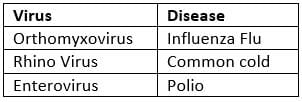RRB JE CE (CBT I) Mock Test- 3 - Question 95

A fog is an aerosol of _______ particles.

Detailed Solution for RRB JE CE (CBT I) Mock Test- 3 - Question 95
• A fog is an aerosol of liquid particles, in particular a low cloud.

• Aerosols are dispersions in gases. In aerosols the particles often exceed the usual size limits for colloids. If the dispersed particles are solid, it is called aerosols of solid particles, if they are liquid, they are called aerosols of liquid particles.

RRB JE CE (CBT I) Mock Test- 3 - Question 96

____________ do not fall under the category of Metals.

Detailed Solution for RRB JE CE (CBT I) Mock Test- 3 - Question 96
Phosphorus is a non-metal. It is a chemical element with symbol P and atomic number 15. Elemental phosphorus exists in two major forms, white phosphorus & red phosphorus. It is an important constituent of bones and teeth, and it is essential to the growth of living organisms.
RRB JE CE (CBT I) Mock Test- 3 - Question 97

The longest cells in the human body are______.

Detailed Solution for RRB JE CE (CBT I) Mock Test- 3 - Question 97
The longest cells in the human body are the nerve cells . The cell bodies of Nerve cells are located in the base of your spinal cord and their axons run down to the ends of your toes. They are approximately 1 meter long.
RRB JE CE (CBT I) Mock Test- 3 - Question 98

What is the scientific name of the peacock?

Detailed Solution for RRB JE CE (CBT I) Mock Test- 3 - Question 98
Pavo cristatus is the scientific name of the peacock.

Lotus - Nelumbo nucifera

Rice - Orzya Sativa

Tiger - Panthera tigris

RRB JE CE (CBT I) Mock Test- 3 - Question 99

What is the largest source of power in India?

Detailed Solution for RRB JE CE (CBT I) Mock Test- 3 - Question 99
Thermal power is the "largest" source of power in India. There are different types ofthermal power plants based on the fuel used to generate the steam such as coal, gas, and Diesel. About 71% of electricity consumed in India are generated bythermal power plants.
RRB JE CE (CBT I) Mock Test- 3 - Question 100

Speed of sound is the greatest in _________.

Detailed Solution for RRB JE CE (CBT I) Mock Test- 3 - Question 100
Sound travels faster in liquids and non-porous solids than it does in air. It travels about 4.3 times as fast in water (1,484 m/s), and nearly 15 times as fast in iron (5.120 m/s), than in air at 20 degrees Celsius. Sound waves in solids are composed of compression waves (just as in gases and liquids), but also exhibit a different type of sound wave called a shear wave, which occurs only in solids. The speed of sound is the distance travelled during a unit of time by a sound is the distance travelled during a unit of time by a sound wave propagating through an elastic medium. In dry air at 20°/C (68 °F), the speed of sound is 343.2 meters per second (1,126 ft/s). This is 1,236 kilometers per hour (768 mph), or about one kilometer in three seconds or approximately one mile in five seconds.

## RRB JE Mock Test Series for Civil Engineering (CE)

158 tests
Information about RRB JE CE (CBT I) Mock Test- 3 Page
In this test you can find the Exam questions for RRB JE CE (CBT I) Mock Test- 3 solved & explained in the simplest way possible. Besides giving Questions and answers for RRB JE CE (CBT I) Mock Test- 3, EduRev gives you an ample number of Online tests for practice

## RRB JE Mock Test Series for Civil Engineering (CE)

158 tests(Scan QR code)